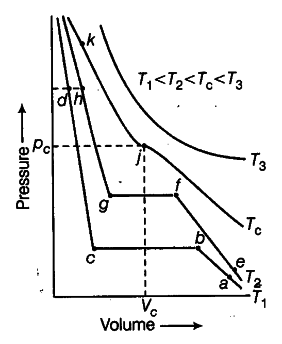# Isotherms of carbon dioxide at various temperatures are represented in the figure

Isotherms of carbon dioxide at various temperatures are represented in the figure. Answer the following questions based on the figure.
(i) In which state will CO_{ 2 } exist between the points a and b at - temperature T_{ 1 }
(if) At what point will CO_{ 2 } start liquefying when temperature is T_{ 1 }
(iii) At what point will CO_{ 2 } be completely liquefied when temperature is T_{ 2 }
(iv) Will condensation take place when the temperature is T_{ 3 }?
(v) What portion of the isotherm at T_{ 1 } represent liquid and gaseous CO_{ 2 } at equilibrium?(i) \$CO_{ 2 }\$ will exist as gaseous state between ‘a’ and ‘b’.
(ii) At point ‘b’, \$CO_{ 2 }\$ will start liquefying.
(iii) At point ‘g’, \$CO_{ 2 }\$ will be completely liquefied.
(iv) No, because \$T_{ 3 }\$ is greater than \$T_{ c }\$ (critical temperature).
(v) Between 'b’ and ‘c is the portion of isotherm at which liquid \$CO_{ 2 }\$ is in equilibrium with gaseous \$CO_{ 2 }\$